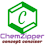Welcome to Chem Zipper.com......: A mixture of weighing 228 g contain CaCl2 and NaCl . If this mixture is dissolved in 10 kg of water and form ideal solution that boil at 100.364 ℃ The mol % of NaCl in mixture is [ kb of water = 0.52 K per mol Kg]

## Saturday, May 16, 2020

### A mixture of weighing 228 g contain CaCl2 and NaCl . If this mixture is dissolved in 10 kg of water and form ideal solution that boil at 100.364 ℃ The mol % of NaCl in mixture is [ kb of water = 0.52 K per mol Kg]

Given that 228 g mixture of NaCl and CaCl2 consider that “x” g NaCl and (228-x) gm CaCl2 present in this mixture:

NaCl and CaCl2 both are strong electrolytes hence Vant’s Hoff factor are 2 and 3 respectively.

Moles of NaCl = x/58.5

Moles of CaCl2 = 228-x

And hence molality of NaCl and CaCl2 are

Molality of NaCl = ×/58.5×10kg

Molality of CaCl2= 228-x/111×10kg

We know that

∆Tb = i1m1+i2m2

100.364= 2(×/58.5×10kg) +3(228-x/111×10kg)

On solving we got x= 117 g

Moles of NaCl= 117/58.5 =2

Moles of CaCl2= 111/111=1

Mole percentage (%) 0f NaCl = (2/2+1)x100= 66.67%

Related Questions:

### (6) A saturared solution of XCl3 has a vapour pressure 17.20 mm Hg at 20°C, while pure water vapour pressure is 17.25 mm Hg. Solubility product (Ksp) of XCl3 at 20°C is

1.Finally got it !!

1.Great..well done

2.Looking for this answer all over the internet

1.3.